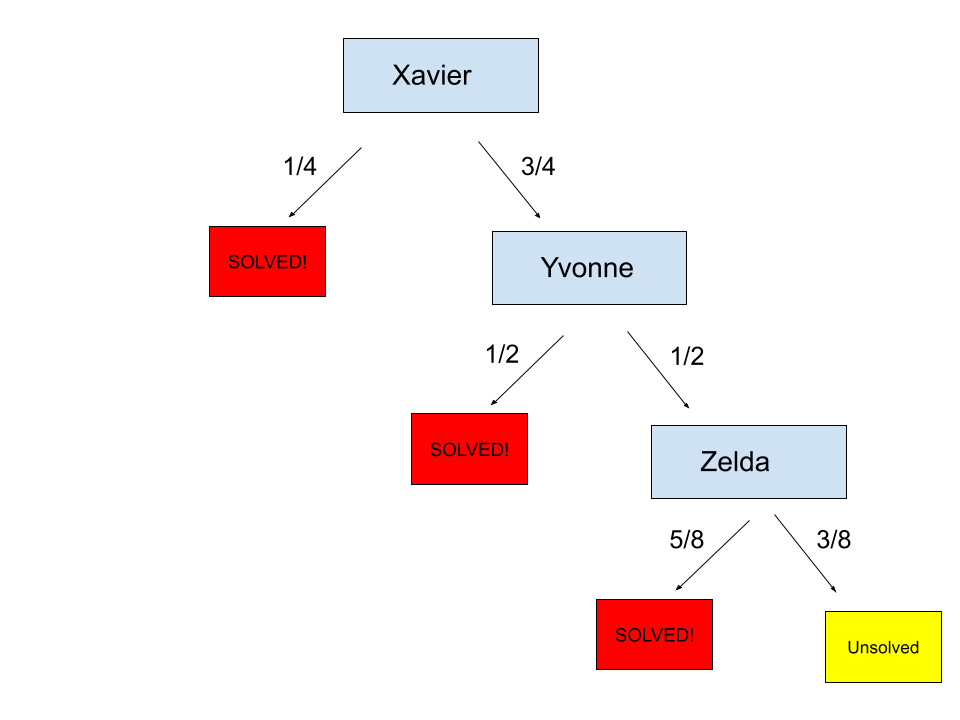By: Rich Zwelling, Apex GMAT Instructor
Date: 11th March, 2021

In our last post, we discussed a solution for the following question, which is a twist on an Official Guide GMAT probability problem:

Xavier, Yvonne, and Zelda individual probabilities for success on a certain problem are 1/4, 1/2 and 5/8, respectively. Xavier will attempt the problem first. If he solves it, Yvonne and Zelda will not attempt it. If Xavier cannot solve it, Yvonne will attempt it next. If she solves it, Zelda will not attempt it. If Yvonne cannot solve it, Zelda will then attempt it. What is the probability that Zelda does not get to attempt the problem?

A) 3/16
B)
5/8
C) 3/8
D) 5/64
E) 3/64

We also mentioned that there was an alternate way to solve it. Did you find it? In truth, it relates to something we discussed in a previous post we did on GMAT Combinatorics, specifically Combinations with Restrictions. In that post, we discussed the idea of considering combinations in which you’re not interested. It might seem counterintuitive, but if you subtract those out from the total number of combinations possible, you’re left with the number of combinations in which you are interested:

You can actually do something similar with probability. Take the following basic example:

Suppose I told you to flip a fair coin five times, “fair” meaning that it has an equal chance of landing heads-up or tails-up. I then wanted to know the probability that I flip at least one head. Now, when you think about it, the language “at least one” involves so many desired possibilities here. It could be 1 head, 2 heads, …, all the way up to 5 heads. I’d have to calculate each of those probabilities individually and add them up.

Or…

I could consider what is not desired, since the possibilities are so much fewer:

All of the above must add to 100% or 1, meaning all possible outcomes. So why not figure out the probability that I get 0 heads (or all tails), and then subtract it from 100% or 1 (depending on whether I’m using a percentage or decimal/fraction)? I’ll then be left with all the possibilities in which I’m actually interested, without the need to do any more calculations.

Each time I flip the coin, there is a ½ chance that I flip a tail. This is the same each of the five times I flip the coin. I then multiply all of the probabilities together:

½ x ½ x ½ x ½ x ½ = 1 / 25  = 1 / 32

Another way to view this is through combinatorics. Remember, probability is always Desired outcomes / Total possible outcomes. If we start with the denominator, there are two outcomes each time we flip the coin. That means for five flips, we have 25 or 32 possible outcomes, as illustrated here with our slot method:

_2_  _2_  _2_  _2_  _2_ = 32

Out of those 32 outcomes, how many involve our (not) desired outcome of all tails? Well, there’s only one possible way to do that:

_T_  _T_  _T_  _T_  _T_    ← Only 1 outcome possible

It really is that straightforward: with one outcome possible out of 32 total, the probability is 1/32 that we flip all tails.

Now remember, that is our, not desired. Our desired is the probability of getting at least one head

So, since the probability of getting 0 heads (all tails) is 1/32, we simply need to subtract that from 1 (or 32/32) to get our final result. The probability that we flip at least one head if we flip a fair coin five times is 31/32.

#### Application to problem from previous post

So now, how do we work that into the problem we did last time? Well, in the previous post, we took a more straightforward approach in which we considered the outcomes we desired. But can we use the above example and consider not desired instead? Think about it and give it a shot before reading the explanation:

Xavier, Yvonne, and Zelda individual probabilities for success on a certain problem are 1/4, 1/2 and 5/8, respectively. Xavier will attempt the problem first. If he solves it, Yvonne and Zelda will not attempt it. If Xavier cannot solve it, Yvonne will attempt it next. If she solves it, Zelda will not attempt it. If Yvonne cannot solve it, Zelda will then attempt it. What is the probability that Zelda does not get to attempt the problem?

A) 3/16
B) 5/8
C) 3/8
D) 5/64
E) 3/64

#### Explanation

In this question, our desired case is that Zelda does not attempt the problem. That means, quite simply, that our not desired case is that Zelda does get to attempt it. This requires us analytically to consider how such a case would arise. Let’s map out the possibilities with probabilities:Notice that two complementary probabilities are presented for each box. For example, since there is a 1/4 chance Xavier solves the problem (left arrow), we include the 3/4 probability that he does not solve the problem (right arrow).

If Zelda does get to attempt it, it’s clear from the above that first Xavier and Yvonne must each not solve it. There is a 3/4 and a 1/2 chance, respectively, of that happening. This is also a dependent situation. Xavier must not solve AND Yvonne must not solve. Therefore, we will multiply the two probabilities together to get ¾ x ½ = ⅜. So there is a 3/8 chance of getting our not desired outcome of Zelda attempting the problem.

So, we can finally subtract this number from 1 (or 8/8) and see that there is a 5/8 chance of Zelda not getting to attempt the problem. The correct answer is B.

Next time, we’ll discuss how GMAT Probability and Combinatorics can combine to form some interesting problems…